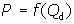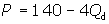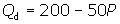3.2 Demand

How can you increase the demand for your company's product? How will your competitor's production and pricing decisions affect the demand for your product? How will raising the price of your product by \$20 affect your sales? What determines the prices of the inputs you need to produce your products? Should you expect these input prices to rise or fall in the near future?

To answer important questions like these, you need to understand the economic meaning of the demand for a good. Organisations that understand demand and the factors that influence demand can respond optimally to changes in the demand for their products or the products they rely on as inputs.

# Law of Demand

The demand for a good refers to the relation between the price of a good and the quantity of the good that consumers will be willing and able to purchase at various prices, holding all other factors that affect purchase decisions constant.

The law of demand states that other things being equal, as the price of a good increases, the quantity demanded of that good decreases.

This is true both for the individual consumer's demand and the market demand for a good, because the market consists of many individual consumers.

For example, suppose that through a combination of market research and data from pricing experiments, a manager at Ekina Sports estimates that at a price of \$100 per pair, consumers would purchase, or "demand", 10,000 pairs of Ekina running shoes each week. The manager further estimates that consumers would demand 15,000 pairs per week if the price were decreased to \$80, and 20,000 pairs per week if the price were further decreased to \$60.

If you assume that the relation between price (P) and quantity demanded (Qd) continues in a similar fashion for even higher or lower prices, you can illustrate the demand for Ekina shoes on a graph.

Start by constructing a demand schedule that lists various prices and the quantity of shoes consumers would demand at each price. For example, assume Ekina further estimates that at a price of \$140, consumers would not demand any shoes; at a price of \$120, consumers would demand five thousand pairs; at a price of \$100, consumers would demand ten thousand pairs, and so on.Then draw x- and y-axes and show price (P) on the y-axis and the quantity of shoes demanded (Q) on the x-axis.

You can plot the price-quantity pairs (from the demand schedule) on the graph and connect them in a linear fashion to produce the demand line, or "market demand curve" as it is more generally known.As you look at the graph, notice how the line slopes downwards, indicating that as the price of shoes decreases, the quantity demanded increases. Consumers are willing and able to purchase more shoes at the lower prices than they were willing and able to purchase at the higher prices.

The following link provides a brief look at how the law of demand works for energy.

Observing the Law of Demand

The law of demand is not necessarily something that applies only for explicit dollar prices. Click on the link below for another possibility.

The Law of Demand in Different Environments

# Demand Function

It is often convenient to express this relation between price and quantity demanded as a mathematical function. You can write the function in two equivalent ways. One way expresses price as a function of quantity demanded:The second way expresses quantity demanded as a function of price:You read the function above: "quantity demanded is a function of price," not, "quantity demanded equals f times P." Quantity demanded depends on price; it is the dependent variable. P is the independent variable.

What does the demand function tell you?

The following linear function summarises the relation between price and quantity demanded represented by the Demand Schedule and Market Demand Curve above:For a reminder of what linear functions and their properties are, click on the link below.

Notice how this function reflects the information in the graph below. For example, substituting a quantity of ten thousand pairs of shoes for Qd results in a price of \$100, which indicates that consumers would demand ten thousand pairs of shoes at a price of \$100 per pair.You can express the same relation between price and quantity demanded with quantity demanded on the left side of the function. Rearranging the same equation to isolate quantity demanded yields:

Q = 35 - P/4

Notice how the conversion maintains the underlying relation between price and quantity demanded. Substituting \$100 for price results in Qd = 10 (thousand) as before.

The function

Qd = 35 - P/4

is formally called a demand function because it expresses quantity demanded as a function of price.

The functionis called the inverse demand function because it represents the same demand relationship, just inverted. Because both equations represent the same demand relationship, many economists refer to either as just the demand function.

You will often use the demand function with price on the left side because it is consistent with the way economists represent demand graphically. That is, it reflects the placement of price on the y-axis and quantity demanded on the x-axis. This subject will adhere to this practice throughout.

Generally, you can express the demand function for any linear demand as

a = the y-intercept

-b = the slope of the function

Notice that a corresponds to the price at which quantity demanded becomes zero, and b indicates the change in price that will generate a one-unit change in quantity demanded. In practice, you will often estimate these parameters using the statistical technique regression analysis. Regression analysis involves collecting real observations on two variables, such as P and Q, and then using these observations to estimate a mathematical relation between the two variables.

If you want to review the mathematics of constants, variables and coefficients, click on the link below.

Analytical Methods: Constants, Variables and Coefficients

The information contained in a simple demand equation should not be underestimated. You will find later in the subject that you can derive a wealth of additional information from a simple demand equation.

Example: Demand for coffee
Assume a bakery finds the daily demand function for its coffee to beP = price per cup of coffee

Qd = the number of cups that consumers would be willing to buy per day at each price

To plot this demand function on a graph, you first want to solve the demand function for P. Expressing demand in terms of price is consistent with placing price on the y-axis and quantity demanded on the x-axis.The demand function above has a slope of -0.02, and a y-intercept of 4. By substituting values for Qd, such as 50 and 100, you can find two additional quantity-price pairs (50, \$3; 100, \$2). You can now plot the pairs, or points, on a graph, with price on the y-axis and quantity demanded on the x-axis.Click on the following link for another example of how to draw a linear demand curve.

Example of a Linear Demand Curve

Sometimes it is better to represent a demand function in a nonlinear form. Before doing this, you might like to review the mathematics of non-linear functions in the following link.

Analytical Methods: Nonlinear Functions

You can take a look at the advanced material in the following link to explore nonlinear demand functions.

(Advanced) Nonlinear Demand Functions

# Other Factors That Influence Demand

Recall that the law of demand states that other things being equal, as the price of a good increases, the quantity demanded of that good decreases. But what if one of the "other things" changes? For example, how would an economic recession affect the demand for Ekina shoes? How would an increased marketing campaign by Ekina affect the demand for its shoes? What would happen in July if Ekina announced that it would be raising all of its shoe prices in September in response to increased costs of rubber and other materials? The demand relationship in each of these cases would change.

When an important factor other than the price of a good itself changes, a change in demand for that good may occur. In the Ekina shoes example, an increase in demand means that consumers are now willing to purchase more shoes at each price. Graphically, an increase in demand appears as a rightward (or "upward") shift of the demand curve.Similarly, a decrease in demand for Ekina shoes means that consumers are willing to purchase fewer shoes at each price than previously. Graphically, a decrease in demand appears as a leftward (or "downward") shift of the demand curve.Mathematically, an increase or a decrease in demand appears as an increase or a decrease in the y-intercept a, respectively:

## Change in quantity demanded vs. change in demand

A change in quantity demanded occurs when the price of the good alone changes. A change in demand results from a change in one of the factors other than price. When price alone changes, no "shift" occurs in the underlying relationship, you simply move to a higher or lower price-quantity pair along the same demand curve.

The primary factors that can cause a change in demand (or a shift in the demand curve) are

·         consumer income

·         prices of related goods

·         advertising and consumer preferences

·         population

·         seasonal factors

·         expectations

Understanding these factors will allow you to predict the market response to real economic events.

Test your knowledge now with an example of a bakery. Click here to begin.

## Advertising and consumer preferencesIf consumer preferences change so that consumers get more fulfilment from consuming a good, whether the result of a firm's advertising or outside forces, demand for the good will increase. Demand for Ekina's running shoes might increase if Ekina ran commercials in which Olympic track stars endorsed its shoes, or if a medical report announced some new health benefits from jogging.

The link here discusses how changes in consumer preferences impacted upon the demand for energy.

## Expectations Finally, if consumers' expectations about the price of a good changes, demand for the good will change. For instance, if consumers expect prices to rise in the near future, they may demand more of a good today. The opposite is true if consumers expect prices to fall in the future. Homebuyers may delay home purchases if they expect the housing market to be more attractive in a year.

The interactive exercise here allows you to see how each of these factors affects the demand curve for another market - sports drinks.

Topic Summary

In this topic, you have learnt how to

• explain the law of demand and why demand curves slope downwards
• express demand functions mathematically and graphically
• identify how changes in consumer income, prices of related good, advertising and consumer preferences, population, seasonal factors and expectations can cause changes in demand

Now go on to topic 3.3, “Price Elasticity”.